A cyclic process for 1 mole of an ideal gas is shown in figure in the V-T, diagram. The work done in AB, BC and CA respectively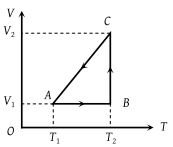(1) $0,\text{\hspace{0.17em}}R{T}_{2}\mathrm{ln}\left(\frac{{V}_{1}}{{V}_{2}}\right)\text{\hspace{0.17em}},\text{\hspace{0.17em}}R\text{\hspace{0.17em}}\left({T}_{1}-{T}_{2}\right)$

(2) $R\left({T}_{1}-{T}_{2}\right),\text{\hspace{0.17em}}0,\text{\hspace{0.17em}}R{T}_{1}\mathrm{ln}\frac{{V}_{1}}{{V}_{2}}$

(3) $0,\text{\hspace{0.17em}}R{T}_{2}\mathrm{ln}\left(\frac{{V}_{2}}{{V}_{1}}\right)\text{\hspace{0.17em}},\text{\hspace{0.17em}}R\text{\hspace{0.17em}}\left({T}_{1}-{T}_{2}\right)$

(4) $0,\text{\hspace{0.17em}}R{T}_{2}\mathrm{ln}\left(\frac{{V}_{2}}{{V}_{1}}\right)\text{\hspace{0.17em}},\text{\hspace{0.17em}}R\text{\hspace{0.17em}}\left({T}_{2}-{T}_{1}\right)$

High Yielding Test Series + Question Bank - NEET 2020

Difficulty Level:

A cyclic process ABCD is shown in the P-V diagram. Which of the following P-T curves represent the same process ?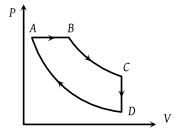(1)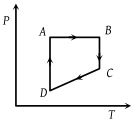(2)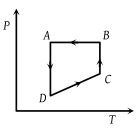(3)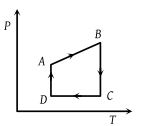(4)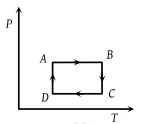Concept Questions :-

Types of processes
High Yielding Test Series + Question Bank - NEET 2020

Difficulty Level:

Carnot cycle (reversible) of a gas represented by a Pressure-Volume curve is shown in the diagram . Consider the following statements

I. Area ABCD = Work done on the gas

II. Area ABCD = Net heat absorbed

III. Change in the internal energy in cycle = 0

Which of these are correct ?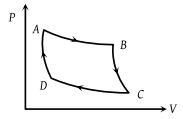(1) I only

(2) II only

(3) II and III

(4) I, II and III

Concept Questions :-

First law of thermodynamics
High Yielding Test Series + Question Bank - NEET 2020

Difficulty Level:

The temperature-entropy diagram of a reversible engine cycle is given in the figure. Its efficiency is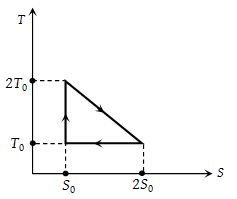(1) 1/3

(2) 2/3

(3) 1/2

(4) 1/4

Concept Questions :-

Heat engine and refrigerator
High Yielding Test Series + Question Bank - NEET 2020

Difficulty Level:

Work done in the given P-V diagram in the cyclic process is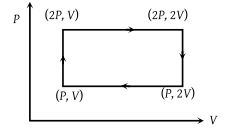(1) PV

(2) 2PV

(3) PV/2

(4) 3PV

Concept Questions :-

Work done by gas
High Yielding Test Series + Question Bank - NEET 2020

Difficulty Level:

A cyclic process ABCA is shown in the V-T diagram. Process on the P-V diagram is -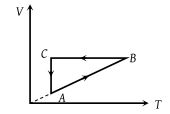(1)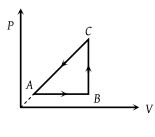(2)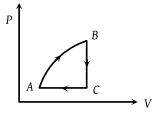(3)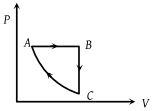(4)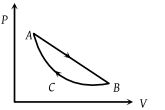Concept Questions :-

Types of processes
High Yielding Test Series + Question Bank - NEET 2020

Difficulty Level:

In the figure given two processes A and B are shown by which a thermo-dynamical system goes from initial to final state F. If ΔQA and ΔQB are respectively the heats supplied to the systems then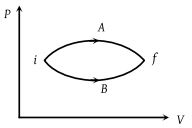(1) ΔQA = ΔQB

(2) $\Delta {Q}_{A}\ge \Delta {Q}_{B}$

(3) ΔQA < ΔQB

(4) ΔQA > ΔQB

Concept Questions :-

First law of thermodynamics
High Yielding Test Series + Question Bank - NEET 2020

Difficulty Level:

In the cyclic process shown in the figure, the work done by the gas in one cycle is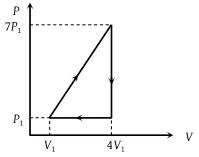(1) 28 P1V1

(2) 14 P1V1

(3) 18 P1V1

(4) 9 P1V1

Concept Questions :-

Work done by gas
High Yielding Test Series + Question Bank - NEET 2020

Difficulty Level:

Heat energy absorbed by a system in going through a cyclic process shown in figure is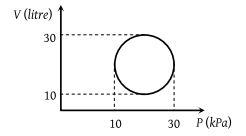(1) 107 π J

(2) 104 π J

(3) 102π J

(4) 10–3π J

Concept Questions :-

Cyclic process
High Yielding Test Series + Question Bank - NEET 2020

Difficulty Level:

A thermodynamic system is taken from state A to B along ACB and is brought back to A along BDA as shown in the PV diagram. The net work done during the complete cycle is given by the area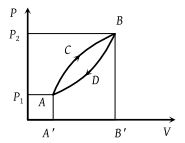(1) P1ACBP2P1

(2) ACBB'A'A

(3) ACBDA